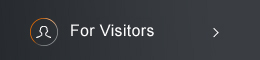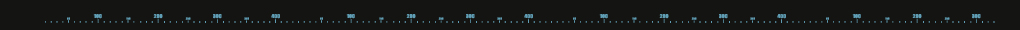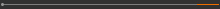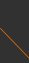Sponsored by[ Events ]

﻿
Activity Search
Sort out
 select all Seminars and Talks Conferences, Workshops and Special Events Courses and Lecture Series ElseField
 select all Number Theory and Representation Theory Algebraic Geometry Differential Geometry and Geometric Analysis Differential Equations and Stochastic Analysis Scientific Computing Interdisciplinary Studies Laboratory of Data Science Harmonic Analysis OthersYear
 select 2015 2016 2017 2018 2019 2020 2021 2022 2023 2024NCTS International Geometric Measure Theory Seminar16:00 - 18:00, September 20, 2023 (Wednesday) HyHyve, Online seminar (線上演講 HyHyve) Rectifiability, Finite Hausdorff Measure, and Compactness for Non-minimizing Bernoulli Free Boundaries Georg Weiss (Universität Duisburg-Essen) Abstract While there are numerous results on minimizers or stable solutions of the Bernoulli problem proving regularity of the free boundary and analyzing singularities, much less is known about critical points of the corresponding energy. Saddle points of the energy (or of closely related energies) and solutions of the corresponding time-dependent problem occur naturally in applied problems such as water waves and combustion theory. For such critical points u–which can be obtained as limits of classical solutions or limits of a singular perturbation problem–it has been open since [Weiss03] whether the singular set can be large and what equation the measure ∆u satisfies, except for the case of two dimensions. In the present result we use recent techniques such as a frequency formula for the Bernoulli problem as well as the celebrated Naber-Valtorta procedure to answer this more than 20 year old question in an affirmative way: For a closed class we call variational solutions of the Bernoulli problem, we show that the topological free boundary ∂{u > 0} (including degenerate singular points x, at which u(x + r·)/r → 0 as r → 0) is countablyHn-1- rectifiable and has locally finite Hn−1-measure, and we identify the measure ∆u completely. This gives a more precise characterization of the free boundary of u in arbitrary dimension than was previously available even in dimension two. We also show that limits of (not necessarily minimizing) classical solutions as wellas limits of critical points of a singularly perturbed energy are variational solutions, so that the result above applies directly to all of them.   This is a joint work with Dennis Kriventsov (Rutgers). Agenda  Get-together (30 min)  Presentation Georg Weiss (60 min)  Questions and discussions (30 min)  Seminar website https://ncts.ntu.edu.tw/gmt-seminar.html(C) 2021 National Center for Theoretical Sciences